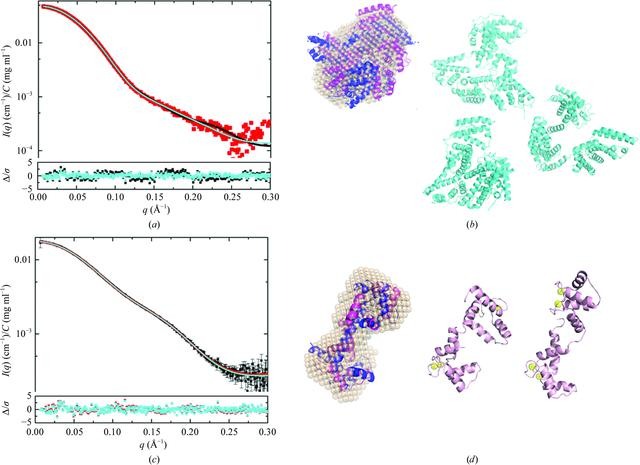disable zoom     view article Figure 3 MultiFoXS modelling results for BSA and CaM. (a) Model fits for BSA: I(q) versus q (red squares) for one-state (black line) and three-state (cyan line) models assuming flexible residues 183–187 and 381–384. The lower inset shows the error-weighted residual difference plots for one-state (black squares) and three-state (cyan squares) models. (b) BSA DAMMIN model (wheat spheres) overlaid with the crystal structure (PDB entry 4f5s, chain A, blue ribbon) and one-state optimized model (magenta ribbon) and representative structures from the three-state optimized model (cyan ribbon models). (c) Model fits to I(q) versus q for CaM: I(q) versus q (black squares) for one-state (red line) and two-state (cyan line) models assuming flexible residues 1–3 and 77–81; the lower inset shows the error-weighted residual difference plots for the one-state (red squares) and two-state (cyan squares) models. (d) CaM DAMMIN model (wheat spheres) overlaid with the crystal structure (PDB entry 1cll, blue ribbon) and the one-state model (magenta ribbon) with the representative two-state models to the right (pink; calcium ions are depicted as yellow spheres). Model overlays were optimized using SUPCOMB (Kozin & Svergun, 2001).STRUCTURALBIOLOGY
ISSN: 2059-7983
Volume 73| Part 9| September 2017| Pages 710-728